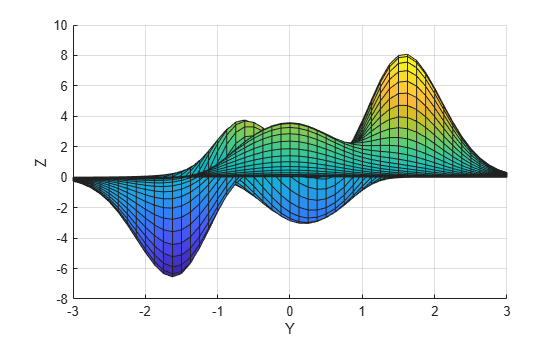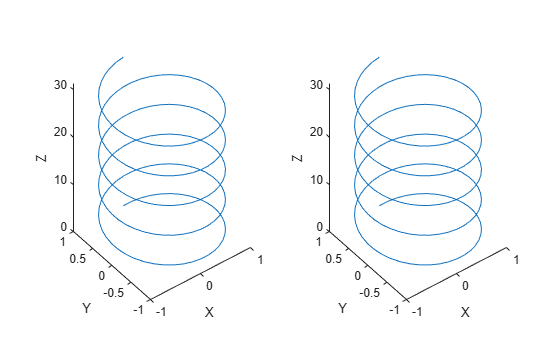# view

Camera line of sight

## Syntax

``view(az,el)``
``view(v)``
``view(dim)``
``view(ax,___)``
``[caz,cel] = view(___)``

## Description

example

````view(az,el)` sets the azimuth and elevation angles of the camera's line of sight for the current axes.```

example

````view(v)` sets the line of sight according to `v`, which is a two- or three-element array: Two-element array — The values are the azimuth and elevation angles respectively.Three-element array — The values are the x-, y-, and z-coordinates of a vector that starts at the center of the plot box and points toward the camera. MATLAB® calculates the azimuth and elevation angles using a unit vector pointing in the same direction. ```

example

````view(dim)` uses the default line of sight for 2-D or 3-D plots. Specify `dim` as `2` for the default 2-D view or `3` for the default 3-D view.```

example

````view(ax,___)` specifies the target axes when changing the line of sight.```

example

````[caz,cel] = view(___)` returns the azimuth and elevation angles as `caz` and `cel`, respectively. Specify input arguments from any of the previous syntaxes to get the angles for the new line of sight. Or, specify no input arguments to get the angles for the current line of sight.```

## Examples

collapse all

Use the `peaks` function to get the x-, y-, and z-coordinates of a surface. Then plot the surface and label each axis.

```[X,Y,Z] = peaks; surf(X,Y,Z) xlabel('X') ylabel('Y') zlabel('Z')```View the plot using an azimuth of `90` degrees and an elevation of `0` degrees. The new line of sight is along the x-axis.

`view(90,0)`Use the `peaks` function to get the x-, y-, and z-coordinates of a surface. Then plot the surface and label each axis.

```[X,Y,Z] = peaks; surf(X,Y,Z) xlabel('X') ylabel('Y') zlabel('Z')```Display the plot in a 2-D view.

`view(2)`Create a set of x-, y-, and z-coordinates and use them to plot a surface. Then label each axis.

```[X,Y] = meshgrid(-5:.5:5); Z = Y.*sin(X) - X.*cos(Y); surf(X,Y,Z) xlabel('X') ylabel('Y') zlabel('Z')```Get the azimuth and elevation angles for this plot.

`[caz,cel] = view`
```caz = -37.5000 ```
```cel = 30 ```

Change the view by specifying `v` as the x- y- and z-coordinates of a vector, and return the new azimuth and elevation angles. The new angles are based on a unit vector pointing in the same direction as `v`.

```v = [-5 -2 5]; [caz,cel] = view(v)``````caz = -68.1986 ```
```cel = 42.8760 ```

Starting in R2019b, you can display a tiling of plots using the `tiledlayout` and `nexttile` functions. Call the `tiledlayout` function to create a 1-by-2 tiled chart layout. Call the `nexttile` function to create the axes objects `ax1` and `ax2`. Use them to create separate but identical line plots.

```t = 0:pi/20:10*pi; xt1 = sin(t); yt1 = cos(t); tiledlayout(1,2) % Left plot ax1 = nexttile; plot3(ax1,xt1,yt1,t) xlabel('X') ylabel('Y') zlabel('Z') % Right plot ax2 = nexttile; plot3(ax2,xt1,yt1,t) xlabel('X') ylabel('Y') zlabel('Z')```Change the view of the right plot to a side view along the x-axis.

`view(ax2,[90 0]);`## Input Arguments

collapse all

Azimuth, specified as an angle in degrees from the negative y-axis. Increasing this angle corresponds to counterclockwise rotation about the z-axis when viewing the x-y plane from above.

The default value depends on whether your chart is in a 2-D or 3-D view. For 2-D charts, the default value is `0`. For 3-D charts, the default value is `-37.5`.

Example: `view(45,25)` sets the azimuth to `45` degrees and the elevation to `25` degrees.

Elevation, specified the minimum angle in degrees between the line of sight and the x-y plane. Increasing the elevation from `-90` to `90` degrees corresponds to a rotation from the negative z-axis to the positive z-axis.

The default value depends on whether your chart is in a 2-D or 3-D view. For 2-D charts, the default value is `90`. For 3-D charts, the default value is `30`.

Example: `view(45,25)` sets the azimuth to `45` degrees and the elevation to `25` degrees.

Line-of-sight vector, specified as one of the following:

• A two-element array containing the azimuth and elevation angles respectively.

• A three-element array containing the x-, y-, and z-coordinates of a vector that originates from the center of the plot box and points toward the camera. MATLAB calculates the azimuth and elevation from a unit vector pointing in the same direction. Thus, the magnitude of the `v` has no effect on the line of sight.Example: `view([45 25])` sets the azimuth to `45` degrees and the elevation to `25` degrees.

Example: `view([20 25 5])` sets the line of sight to a vector that points in the same direction as the vector `[20 25 5]`.

Dimensions, specified as `2` or `3`. This argument sets the azimuth and elevation to the default values that MATLAB uses for a 2-D or 3-D plot.

Value of `dim`AzimuthElevation
`2`
`0``90`
`3`
`-37.5``30`

Target axes, specified as an `Axes` object.

collapse all

### Line of Sight

The line of sight starts at the center of the plot box and points toward the camera. MATLAB defines this line using two angles, the azimuth and the elevation. These angles are measured within a 3-D coordinate system that has its origin at the center of the plot box.

• Azimuth — The angle of rotation around the z-axis, as measured from the negative y-axis. Increasing this angle corresponds to counterclockwise rotation about the z-axis when viewing the x-y plane from above.

• Elevation — The minimum angle between the line of sight and the x-y plane. Increasing the elevation from `-90` to `90` degrees corresponds to a rotation from the negative z-axis to the positive z-axis.## Tips

The `view` function controls the direction of the line of sight, but it does not control the location that the line starts from, nor the camera position. For more control, use the camera functions such as `campos`, `camtarget`, `camup`, `camva`, `camroll`, and `camproj`.